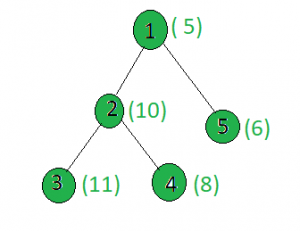# Find the node whose absolute difference with X gives maximum value

• Last Updated : 12 Apr, 2021

Given a tree, and the weights of all the nodes and an integer x, the task is to find a node i such that |weight[i] – x| is maximum.
Examples:

Input:x = 15
Output:
Node 1: |5 – 15| = 10
Node 2: |10 – 15| = 5
Node 3: |11 -15| = 4
Node 4: |8 – 15| = 7
Node 5: |6 -15| = 9

Approach: Perform dfs on the tree and keep track of the node whose weighted absolute difference with x gives the maximum value.
Below is the implementation of the above approach:

## C++

 `// C++ implementation of the approach` `#include ` `using` `namespace` `std;`   `int` `maximum = INT_MIN, x, ans;`   `vector<``int``> graph;` `vector<``int``> weight(100);`   `// Function to perform dfs to find` `// the maximum value` `void` `dfs(``int` `node, ``int` `parent)` `{` `    ``// If current value is more than` `    ``// the current maximum` `    ``if` `(maximum < ``abs``(weight[node] - x)) {` `        ``maximum = ``abs``(weight[node] - x);` `        ``ans = node;` `    ``}` `    ``for` `(``int` `to : graph[node]) {` `        ``if` `(to == parent)` `            ``continue``;` `        ``dfs(to, node);` `    ``}` `}`   `// Driver code` `int` `main()` `{` `    ``x = 15;`   `    ``// Weights of the node` `    ``weight = 5;` `    ``weight = 10;` `    ``weight = 11;` `    ``weight = 8;` `    ``weight = 6;`   `    ``// Edges of the tree` `    ``graph.push_back(2);` `    ``graph.push_back(3);` `    ``graph.push_back(4);` `    ``graph.push_back(5);`   `    ``dfs(1, 1);`   `    ``cout << ans;`   `    ``return` `0;` `}`

## Java

 `// Java implementation of the approach` `import` `java.util.*;`   `class` `GFG` `{`   `static` `int` `maximum = Integer.MIN_VALUE, x, ans; `   `static` `Vector> graph=``new` `Vector>(); ` `static` `Vector weight=``new` `Vector(); `   `// Function to perform dfs to find ` `// the maximum value ` `static` `void` `dfs(``int` `node, ``int` `parent) ` `{ ` `    ``// If current value is more than ` `    ``// the current maximum ` `    ``if` `(maximum < Math.abs(weight.get(node) - x)) ` `    ``{ ` `        ``maximum = Math.abs(weight.get(node) - x); ` `        ``ans = node; ` `    ``} ` `    ``for` `(``int` `i = ``0``; i < graph.get(node).size(); i++) ` `    ``{ ` `        ``if` `(graph.get(node).get(i) == parent) ` `            ``continue``; ` `        ``dfs(graph.get(node).get(i), node); ` `    ``} ` `} `   `// Driver code ` `public` `static` `void` `main(String args[])` `{ ` `    ``x = ``15``; `   `    ``// Weights of the node ` `    ``weight.add(``0``); ` `    ``weight.add(``5``); ` `    ``weight.add(``10``);; ` `    ``weight.add(``11``);; ` `    ``weight.add(``8``); ` `    ``weight.add(``6``); ` `    `  `    ``for``(``int` `i = ``0``; i < ``100``; i++)` `    ``graph.add(``new` `Vector());`   `    ``// Edges of the tree ` `    ``graph.get(``1``).add(``2``); ` `    ``graph.get(``2``).add(``3``); ` `    ``graph.get(``2``).add(``4``); ` `    ``graph.get(``1``).add(``5``); `   `    ``dfs(``1``, ``1``); `   `    ``System.out.println( ans); ` `}` `} `   `// This code is contributed by Arnab Kundu`

## Python3

 `# Python implementation of the approach` `from` `sys ``import` `maxsize`   `# Function to perform dfs to find` `# the minimum value` `def` `dfs(node, parent):` `    ``global` `minimum, graph, weight, x, ans`   `    ``# If current value is less than` `    ``# the current minimum` `    ``if` `minimum < ``abs``(weight[node] ``-` `x):` `        ``minimum ``=` `abs``(weight[node] ``-` `x)` `        ``ans ``=` `node`   `    ``for` `to ``in` `graph[node]:` `        ``if` `to ``=``=` `parent:` `            ``continue` `        ``dfs(to, node)`   `# Driver Code` `if` `__name__ ``=``=` `"__main__"``:` `    ``minimum ``=` `-``maxsize` `    ``graph ``=` `[[] ``for` `i ``in` `range``(``100``)]` `    ``weight ``=` `[``0``] ``*` `100` `    ``x ``=` `15` `    ``ans ``=` `0`   `    ``# Weights of the node` `    ``weight[``1``] ``=` `5` `    ``weight[``2``] ``=` `10` `    ``weight[``3``] ``=` `11` `    ``weight[``4``] ``=` `8` `    ``weight[``5``] ``=` `6`   `    ``# Edges of the tree` `    ``graph[``1``].append(``2``)` `    ``graph[``2``].append(``3``)` `    ``graph[``2``].append(``4``)` `    ``graph[``1``].append(``5``)`   `    ``dfs(``1``, ``1``)`   `    ``print``(ans)`   `# This code is contributed by` `# sanjeev2552`

## C#

 `// C# implementation of the approach` `using` `System;` `using` `System.Collections.Generic;` `    `  `class` `GFG` `{`   `static` `int` `maximum = ``int``.MinValue, x, ans; `   `static` `List> graph = ``new` `List>(); ` `static` `List<``int``> weight = ``new` `List<``int``>(); `   `// Function to perform dfs to find ` `// the maximum value ` `static` `void` `dfs(``int` `node, ``int` `parent) ` `{ ` `    ``// If current value is more than ` `    ``// the current maximum ` `    ``if` `(maximum < Math.Abs(weight[node] - x)) ` `    ``{ ` `        ``maximum = Math.Abs(weight[node] - x); ` `        ``ans = node; ` `    ``} ` `    ``for` `(``int` `i = 0; i < graph[node].Count; i++) ` `    ``{ ` `        ``if` `(graph[node][i] == parent) ` `            ``continue``; ` `        ``dfs(graph[node][i], node); ` `    ``} ` `} `   `// Driver code ` `public` `static` `void` `Main(String []args)` `{ ` `    ``x = 15; `   `    ``// Weights of the node ` `    ``weight.Add(0); ` `    ``weight.Add(5); ` `    ``weight.Add(10);; ` `    ``weight.Add(11);; ` `    ``weight.Add(8); ` `    ``weight.Add(6); ` `    `  `    ``for``(``int` `i = 0; i < 100; i++)` `    ``graph.Add(``new` `List<``int``>());`   `    ``// Edges of the tree ` `    ``graph.Add(2); ` `    ``graph.Add(3); ` `    ``graph.Add(4); ` `    ``graph.Add(5); `   `    ``dfs(1, 1); `   `    ``Console.WriteLine( ans); ` `}` `}`   `// This code is contributed by Princi Singh`

## Javascript

 ``

Output:

`1`

Complexity Analysis:

• Time Complexity : O(N).
In dfs, every node of the tree is processed once and hence the complexity due to the dfs is O(N) if there are total N nodes in the tree. Therefore, the time complexity is O(N).
• Auxiliary Space : O(1).
Any extra space is not required, so the space complexity is constant.

My Personal Notes arrow_drop_up
Recommended Articles
Page :